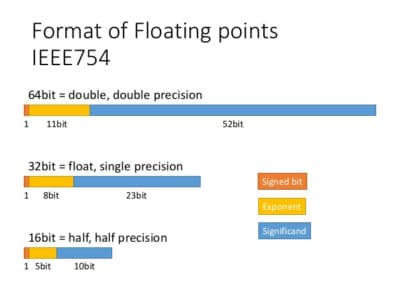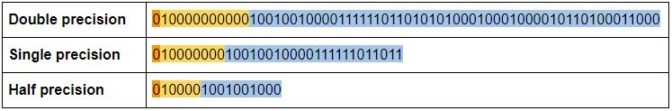# What’s the Difference Between Single-, Double-, Multi- and Mixed-Precision Computing?

by

There are a few different ways to think about pi. As apple, pumpkin and key lime … or as the different ways to represent the mathematical constant of ℼ, 3.14159, or, in binary, a long line of ones and zeroes.

An irrational number, pi has decimal digits that go on forever without repeating. So when doing calculations with pi, both humans and computers must pick how many decimal digits to include before truncating or rounding the number.

In grade school, one might do the math by hand, stopping at 3.14. A high schooler’s graphing calculator might go to 10 decimal places — using a higher level of detail to express the same number. In computer science, that’s called precision. Rather than decimals, it’s usually measured in bits, or binary digits.

For complex scientific simulations, developers have long relied on high-precision math to understand events like the Big Bang or to predict the interaction of millions of atoms.

Having more bits or decimal places to represent each number gives scientists the flexibility to represent a larger range of values, with room for a fluctuating number of digits on either side of the decimal point during the course of a computation. With this range, they can run precise calculations for the largest galaxies and the smallest particles.

But the higher precision level a machine uses, the more computational resources, data transfer and memory storage it requires. It costs more and it consumes more power.

Since not every workload requires high precision, AI and HPC researchers can benefit by mixing and matching different levels of precision. NVIDIA Tensor Core GPUs support multi- and mixed-precision techniques, allowing developers to optimize computational resources and speed up the training of AI applications and those apps’ inferencing capabilities.

## Difference Between Single-Precision, Double-Precision and Half-Precision Floating-Point Format

The IEEE Standard for Floating-Point Arithmetic is the common convention for representing numbers in binary on computers. In double-precision format, each number takes up 64 bits. Single-precision format uses 32 bits, while half-precision is just 16 bits.

To see how this works, let’s return to pi. In traditional scientific notation, pi is written as 3.14 x 100. But computers store that information in binary as a floating-point, a series of ones and zeroes that represent a number and its corresponding exponent, in this case 1.1001001 x 21.In single-precision, 32-bit format, one bit is used to tell whether the number is positive or negative. Eight bits are reserved for the exponent, which (because it’s binary) is 2 raised to some power. The remaining 23 bits are used to represent the digits that make up the number, called the significand.

Double precision instead reserves 11 bits for the exponent and 52 bits for the significand, dramatically expanding the range and size of numbers it can represent. Half precision takes an even smaller slice of the pie, with just five for bits for the exponent and 10 for the significand.

Here’s what pi looks like at each precision level:## Difference Between Multi-Precision and Mixed-Precision Computing

Multi-precision computing means using processors that are capable of calculating at different precisions — using double precision when needed, and relying on half- or single-precision arithmetic for other parts of the application.

Mixed-precision, also known as transprecision, computing instead uses different precision levels within a single operation to achieve computational efficiency without sacrificing accuracy.

In mixed precision, calculations start with half-precision values for rapid matrix math. But as the numbers are computed, the machine stores the result at a higher precision. For instance, if multiplying two 16-bit matrices together, the answer is 32 bits in size.

With this method, by the time the application gets to the end of a calculation, the accumulated answers are comparable in accuracy to running the whole thing in double-precision arithmetic.

This technique can accelerate traditional double-precision applications by up to 25x, while shrinking the memory, runtime and power consumption required to run them. It can be used for AI and simulation HPC workloads.

As mixed-precision arithmetic grew in popularity for modern supercomputing applications, HPC luminary Jack Dongarra outlined a new benchmark, HPL-AI, to estimate the performance of supercomputers on mixed-precision calculations. When NVIDIA ran HPL-AI computations in a test run on Summit, the fastest supercomputer in the world, the system achieved unprecedented performance levels of nearly 550 petaflops, over 3x faster than its official performance on the TOP500 ranking of supercomputers.

## How to Get Started with Mixed-Precision Computing

NVIDIA Volta and Turing GPUs feature Tensor Cores, which are built to simplify and accelerate multi- and mixed-precision computing. And with just a few lines of code, developers can enable the automatic mixed-precision feature in the TensorFlow, PyTorch and MXNet deep learning frameworks. The tool gives researchers speedups of up to 3x for AI training.

The NGC catalog of GPU-accelerated software also includes iterative refinement solver and cuTensor libraries that make it easy to deploy mixed-precision applications for HPC.

For more information, check out our developer resources on training with mixed precision.

## What Is Mixed-Precision Used for?

Researchers and companies rely on the mixed-precision capabilities of NVIDIA GPUs to power scientific simulation, AI and natural language processing workloads. A few examples:

Earth Sciences

• Researchers from the University of Tokyo, Oak Ridge National Laboratory and the Swiss National Supercomputing Center used AI and mixed-precision techniques for earthquake simulation. Using a 3D simulation of the city of Tokyo, the scientists modeled how a seismic wave would impact hard soil, soft soil, above-ground buildings, underground malls and subway systems. They achieved a 25x speedup with their new model, which ran on the Summit supercomputer and used a combination of double-, single- and half-precision calculations.
• A Gordon Bell prize-winning team from Lawrence Berkeley National Laboratory used AI to identify extreme weather patterns from high-resolution climate simulations, helping scientists analyze how extreme weather is likely to change in the future. Using the mixed-precision capabilities of NVIDIA V100 Tensor Core GPUs on Summit, they achieved performance of 1.13 exaflops.

Medical Research and Healthcare

• San Francisco-based Fathom, a member of the NVIDIA Inception virtual accelerator program, is using mixed-precision computing on NVIDIA V100 Tensor Core GPUs to speed up training of its deep learning algorithms, which automate medical coding. The startup works with many of the largest medical coding operations in the U.S., turning doctors’ typed notes into alphanumeric codes that represent every diagnosis and procedure insurance providers and patients are billed for.
• Researchers at Oak Ridge National Laboratory were awarded the Gordon Bell prize for their groundbreaking work on opioid addiction, which leveraged mixed-precision techniques to achieve a peak throughput of 2.31 exaops. The research analyzes genetic variations within a population, identifying gene patterns that contribute to complex traits.

Nuclear Energy

• Nuclear fusion reactions are highly unstable and tricky for scientists to sustain for more than a few seconds. Another team at Oak Ridge is simulating these reactions to give physicists more information about the variables at play within the reactor. Using mixed-precision capabilities of Tensor Core GPUs, the team was able to accelerate their simulations by 3.5x.